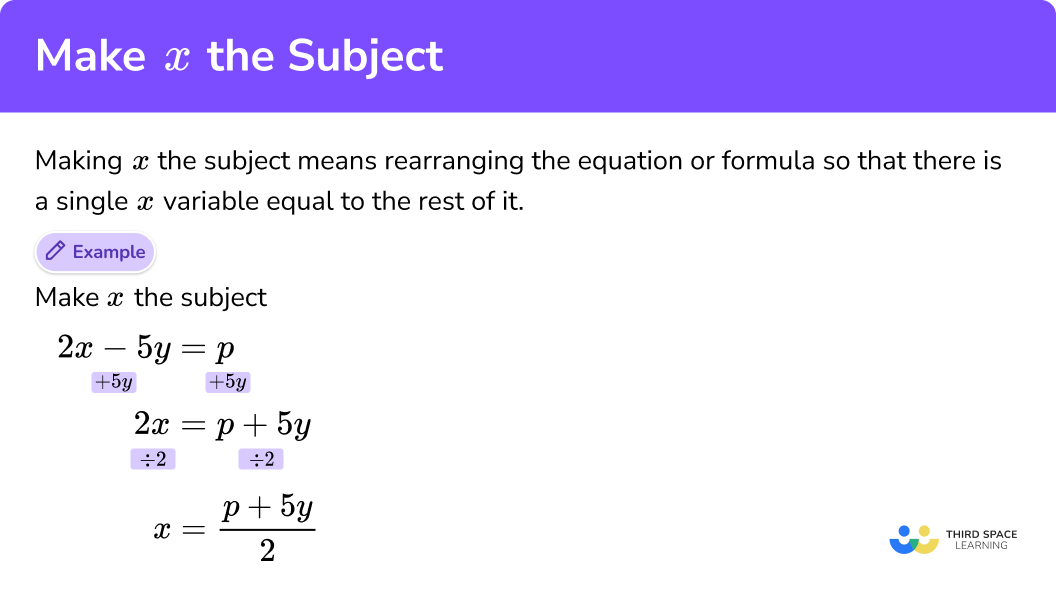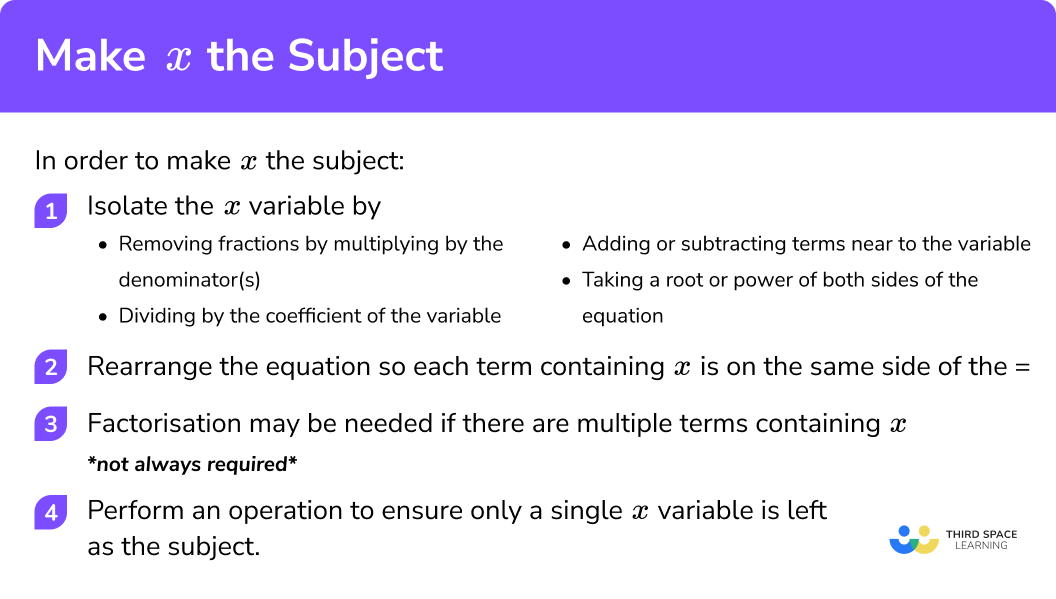# Make x The Subject

Here we will learn about making x the subject of an equation or a formula.

There are also rearranging equations worksheets based on Edexcel, AQA and OCR exam questions, along with further guidance on where to go next if you’re still stuck.

## What does it mean to make x the subject?

Making x the subject of a formula or equation means rearranging the equation or formula so that we have a single x variable equal to the rest of it.

For example,

Make x the subject.

Step-by-step guide: Rearranging equations

### What does it mean to make x the subject?## How to make x the subject

In order to make x the subject:

1. Isolate the variable by:
– Removing any fractions by multiplying by the denominator(s).
– Dividing by the coefficient of the variable.
– Adding or subtracting terms near to the variable.
– Taking a root or power of both sides of the equation.

2. Rearrange the equation so each term containing x is on the same side of the =.

3. Factorisation may be needed if there are multiple terms containing x.

E.g. factorise 2x + 3xy to x(2+3y)
*not always required*

4. Perform an operation to ensure only a single x variable is left as the subject.

### Explain how to make x the subject in 4 steps## Make x the subject examples

### Example 1: multiple step but with single variable

Make x the subject.

Step 1:
Divide each side of the equation by 3

Step 2:
Subtract a from each side of the equation

### Example 2: questions involving x2

Step 1:
Subtract t from both sides of the equation

Step 2:
Square root each side

Remember the square root can be + or \;−

### Example 3: questions involving √x

Step 1:
Add 9a to both sides of the equation

Step 2:
The inverse operation of ‘square root’ is       to ‘square’ each side

Step 3:
The inverse operation of multiply is       divide, so divide both sides by 5

### Example 4: factorisation of the variable is required

Step 1:
Multiply each side of the equation by the denominator

Step 2:
Expand the bracket on the left hand side of the equation and rearrange the equation. This will help us to get all terms with x onto one side of the equation

Step 3:
Factorise the left side of the equation so we have a single variable x.

Step 4: Divide by (a - 5b)
This will leave x as the subject of the equation

### Example 5: factorisation of the variable is required

Step 1:
Multiply each side of the equation by the denominator of the other side.

Step 2:
Expand the bracket on the LHS and RHS of the equation and rearrange. This will help to get all terms with x onto one side of the equation

Step 3:
Factorise the left side of the equation so that we are left with only one of the variable x.

Step 4:
Divide both sides by (b + 8) to leave x as the subject

### Common misconceptions

• Perform the same operation to both sides

When we perform an operation to the left hand side of the equation we have to perform the same operation to the right hand side.

E.g

$\frac{x}{2}=3 y+5$

To isolate the variable x we need to multiply both sides by 2.

$x=6y+5$

This is wrong because we have only multiplied the 3y by 2.

$x=2(3y+5)=6y+10$

This is correct because we have multiplied everything by 2 using brackets.

• Inverse operations

E.g.
To isolate the variable x from 5x we need to perform the inverse operation.

5x = 5 × x, so the inverse operation is divide by 5.

E.g.

$\frac{x}{5}=5 \div x$

so the inverse operation is × 5.

E.g.
To isolate the variable x from x+5 we need to perform the inverse operation:

The inverse operation of +5 is −5.

E.g.
To isolate the variable x from x−5 we need to perform the inverse operation:

The inverse operation of −5 is +5.

• Factorising

To make x the subject there can only be one x visible at the end. We need to get all x’s on one side of the equal sign and then factorise.

E.g.

$x = ax+y$

\begin{aligned} x-a x &=y \\ x(1-a) &=y \\ x &=\frac{y}{1-a} \end{aligned}

• Square rooting a term

When we square rooting a number/variable as an inverse operation the answer can be positive or negative.

E.g.

\begin{align} & {{x}^{2}}=4 \\ & x=\pm \sqrt{4}=\pm 2 \\ \end{align}
$\sqrt{x} \text { should be written as } \pm \sqrt{x}$

### Practice make x the subject questions

1.Make x the subject of the formula.

y = 6(x+8)

x = y – \frac{3}{4}x = \frac{y}{6} + 8x = \frac{y}{6} – 8x = y – 8y = 6(x + 8)

Divide both sides by 6

\frac{y}{6} = x + 8

Then subtract 8 from both sides

x = \frac{y}{6} – 8

2.Make x the subject of the formula.

3p={x}^2-4b

x=\pm\sqrt{3b+4p}x=\pm\sqrt{4b-3p}x=4b+3px=\pm\sqrt{4b+3p}3p={x}^2-4b

3p+4b=x^{2}

Square root both sides

x=\pm\sqrt{4b+3p}

3. Make x the subject of the formula.

6g=\sqrt{7x-8}

x=\frac{6{g}^2+8}{7}x=\frac{36{g}^2-8}{7}x=\frac{36{g}^2+8}{7}x=\frac{6g+8}{7}6g=\sqrt{7x-8}

Square both sides

(6g)^{2}=(\sqrt{7x-8})^{2}

36g^{2}=7x-8

36g^{2}+8=7x

Divide both sides by 7

x=\frac{36{g}^2+8}{7}

4.Make x the subject of the formula.

y=\frac{4x-f}{5x}

x=\frac{f}{5y-4}x=\frac{-f}{5y+4}x=\frac{f}{5y+4}x=\frac{-f}{5y-4}y=\frac{4x-f}{5x}

Multiply both sides by 5x

5xy=4x-f

Subtract 4x from both sides

5xy-4x=-f

Factorise the left hand side

x(5y-4)=-f

Divide both sides by the quantity in the bracket

x=\frac{-f}{5y-4}

5. Make x the subject of the formula.

\frac{y}{3}=\frac{6-2x}{x+3}

x=\frac{18+3y}{y+6}x=\frac{18-3y}{y-6}x=\frac{18-3y}{y+6}x=\frac{18+3y}{y-6}\frac{y}{3}=\frac{6-2x}{x+3}

Multiply each side of the equation by the denominator of the other side.

xy+3y=18-6x

To both sides, add 6x and subtract 3y

xy+6x=18-3y

Factorise the left hand side

x(y+6)=18-3y

Divide by the quantity in the bracket

x=\frac{18-3y}{y+6}

### Make x the subject GCSE Questions

1. Make x the subject of the formula

y=5x-7

(2 marks)

y+7=5x

(1)

\frac{y+7}{5} = x

(1)

2. Make x the subject of the formula

z^{2}=x^{2}-5 a y

(2 marks)

z^{2}+5 a y=x^{2}

(1)

\pm\sqrt{z^{2}+5 a y}=x

(1)

3. Make x the subject of the formula

y=\frac{3(t+5 x)}{x}

(4 marks)

y x=3 t+15 x

(1)

y x-15 x=3 t

(1)

x(y-15)=3 t

(1)

(1)

## Learning checklist

You have now learned how to:

• Understand and use standard mathematical formulae
• Rearrange formulae to change the subject

## Still stuck?

Prepare your KS4 students for maths GCSEs success with Third Space Learning. Weekly online one to one GCSE maths revision lessons delivered by expert maths tutors.

Find out more about our GCSE maths tuition programme.